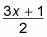##### Linear Algebra For Dummies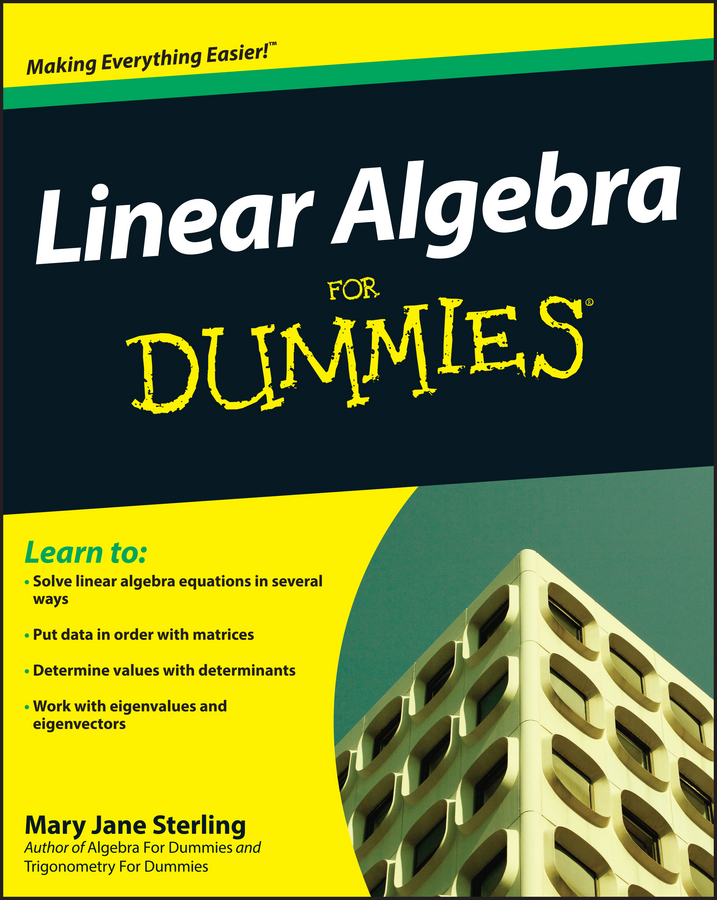If you’re given a function and must find its inverse, first remind yourself that domain and range swap places in the functions. Literally, you exchange f(x) and x in the original equation. When you make that change, you call the new f(x) by its true name — f–1(x) — and solve for this function.

For example, follow the steps to find the inverse of this function: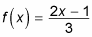1. Switch f(x) and x.

When you switch f(x) and x, you get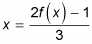(Note: To make the notation less clumsy, you can rewrite f(x) as y and then switch x and y.)

2. Change the new f(x) to its proper name — f–1(x).

The equation then becomes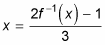3. Solve for the inverse.

This step has three parts:

1. Multiply both sides by 3 to get 3x = 2f–1(x) –1.

2. Add 1 to both sides to get 3x + 1 = 2f–1(x).

3. Lastly, divide both sides by 2 to get your inverse: